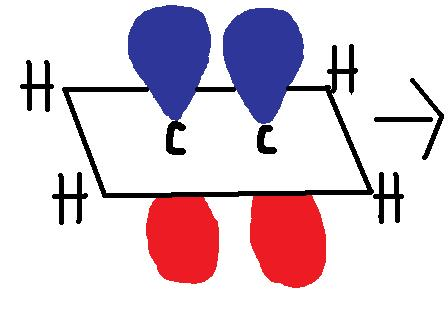# Non-Singular Covalent Bonds

Non-singular covalent bonds are also known as "multiple covalent bonds." There are three types of covalent bonds: single, double, and triple. The name "Non-singular covalent bonds" speaks for itself. Non-singular covalent bonds are covalent bonds that need to share more then one electron pair, so they create double and triple bonds.

## Introduction

The main motive an atom has to bond with other atoms is to fulfill it's need to have eight valence shell electrons (with some exceptions, i.e. Hydrogen). This defines the octet rule. Two different orbital overlaps occur with multiple bonds. The difference between single bonds and multiple bonds is that multiple bonds have one or two pi bonds (one for a double and two for a triple) in addition to the sigma bonds that a single bond creates. The sigma bond with the pi bond is what makes double and triple bonds so strong compared to single bonds. The more bonds there are means there is more overlap between the orbitals. Bond length is also effected by the overlap of the two orbitals, the more overlap the shorter the bond length.

Single Bond= One Sigma bond

Double Bond = One Sigma + One Pi bond

Triple Bond = One Sigma + Two Pi bonds

## Sigma "σ"

A Sigma bond "σ" is the strongest chemical covalent bond. It is created by the "end-to-end" overlap of atomic orbitals. Going more in depth, it is in which the region of electron sharing is along the imaginary line which connects the bonded atoms. They can be formed from two s-orbitals, two p-orbitals, one s- and p- orbital, or with sp hybrid orbitals. The sigma bond is like a cylinder pipe connecting the two orbitals. The two electrons can be found somewhere in the region of space within the sigma bond. The sigma bond is symmetric and can freely rotate around the bond axis.Figure 1. Sigma Bond created from two s atomic orbitals. Used with permission from Wikipedia Commons.

## Pi Bonds "π"

Pi Bonds "π" are created by the "side-to-side" overlapping of two parallel p-orbitals (pictured below). A pi bond is a weaker chemical covalent bond than a sigma bond (since π bonds have a smaller overlap between the orbitals), but when it is put with a sigma bond it creates a much stronger hold between the atoms, thus double and triple bonds are stronger then single bonds.The pi bond looks like two macaroni's sandwiching the sigma bond. Above and below the Pi bonds molecular plane has high electron charge densities. Electrons in a pi bond are sometimes referred to as "Pi electrons." The pi bond is a region of space where you can find the two pi electrons that create the bond. Pi bonds create cis-trans isomers since they prevent free rotation around the bond.

Example of Pi bond formation with ethylene, C2H4 :Figure 2. The two p-orbitals in the first diagram overlap to create the pi bonds.The blue and red color represent the two different phases.

## Delta bonds "δ"

These are where four lobes of one involved electron orbital overlap four lobes of the other involved electron orbital. Of the orbital's node planes, two (and no more), go through both atoms. The Greek letter δ in their name refers to d orbitals, since the orbital symmetry of the delta bond is the same as that of the usual (4-lobed) type of d orbital when seen down the bond axis.

In sufficiently-large atoms, occupied d-orbitals are low enough in energy to participate in bonding. Delta bonds are usually observed in organometallic species. Some ruthenium and molybdenum compounds contain a quadruple bond, which can only be explained by invoking the delta bond. It is possible to excite electrons in acetylene from lower-energy nonbonding orbitals to form a delta bond between the two carbon triple bonds. This is because the orbital symmetry of the pi antibonding orbital is the same as that of the delta bond.Figure 3: delta bond in MO2

## Higher order bonds

Theoretical chemists have conjectured that higher-order bonds (phi bonds and gamma bonds, corresponding to overlap of f and g orbitals) are possible, with even more overlapping lobes of their component atomic orbitals, but no experimental evidence for these has yet been observed.